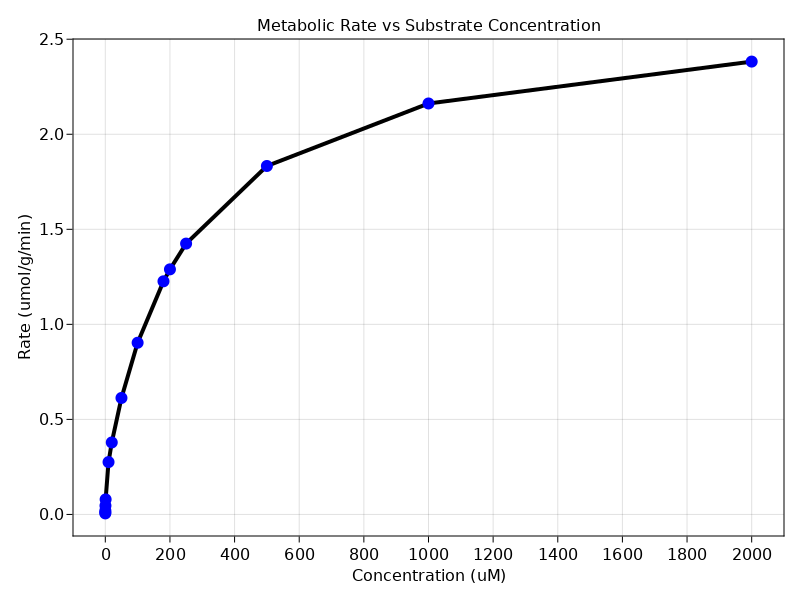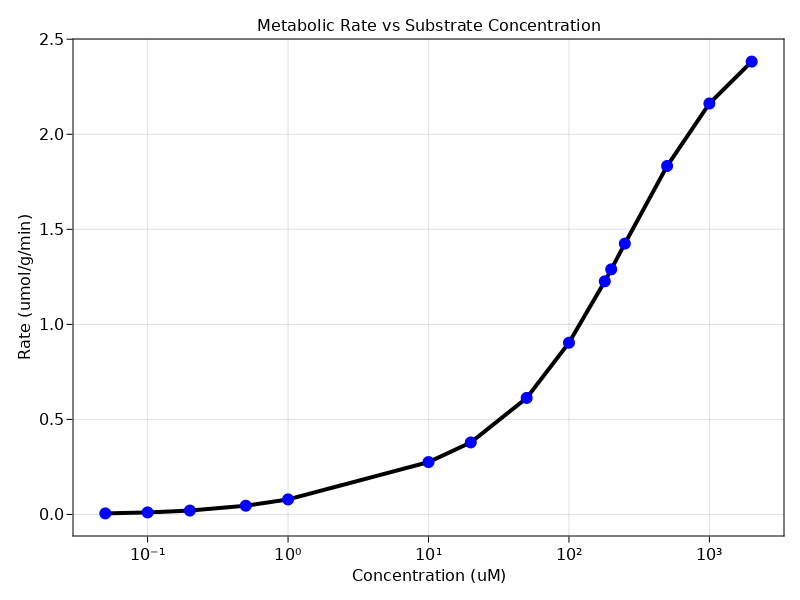# Exercise PK37 - In vitro/In vivo Extrapolation I

### Learning Outcome

In this exercise, you will learn to assess the relationship between rate and concentration using a non-linear clearance model. The Clearance is impacted by two parallel non-linear processes and both of them are taken into account for calculating rates. The use of a single enzyme system was not able to account for the deviations in the system.

### Libraries

Call the "necessary" libraries to get start.

using Random
using Pumas
using PumasUtilities
using CairoMakie
using AlgebraOfGraphics
using DataFramesMeta


### Model

pk_37       = @model begin
desc    = "Invivo/Invitro Extrapolation Model"
end

@param begin
"Maximum Rate of System 1 (μmol/min/g)"
tvvmax1 ∈ RealDomain(lower=0)
"Michaelis-Menten constant for system 1 (μmol/L)"
tvkm1   ∈ RealDomain(lower=0)
"Maximum Rate of System 2 (μmol/min/g)"
tvvmax2 ∈ RealDomain(lower=0)
"Michaelis-Menten constant for system 2 (μmol/L)"
tvkm2   ∈ RealDomain(lower=0)
Ω       ∈ PDiagDomain(4)
end

@random begin
η       ~ MvNormal(Ω)
end

@covariates Conc

@pre begin
Vmax1   = tvvmax1 * exp(η)
Km1     = tvkm1 * exp(η)
Vmax2   = tvvmax2 * exp(η)
Km2     = tvkm2 * exp(η)
_Conc   = Conc
end

@derived begin
rate    = @. ((Vmax1*_Conc/(Km1+_Conc)) + (Vmax2*_Conc/(Km2+_Conc)))
"""
Observed Concentrations (μmol/L)
"""
end
end

PumasModel
Parameters: tvvmax1, tvkm1, tvvmax2, tvkm2, Ω, σ_add
Random effects: η
Covariates: Conc
Dynamical variables:
Derived: rate, dv_rate
Observed: rate, dv_rate


### Parameters

The parameters are as given below. tv represents the typical value for parameters.

• $tvvmax1$ - Maximum Rate of System 1 (μmol/min/g)

• $tvkm1$ - Michaelis-Menten constant for system 1 (μmol/L)

• $tvvmax2$ - Maximum Rate of System 2 (μmol/min/g)

• $tvkm2$ - Michaelis-Menten constant for system 2 (μmol/L)

param = ( tvvmax1 = 2.43386,
tvkm1   = 256.384,
tvvmax2 = 0.22523,
tvkm2   = 2.23625,
Ω       = Diagonal([0.0,0.0,0.0,0.0]),

(tvvmax1 = 2.43386, tvkm1 = 256.384, tvvmax2 = 0.22523, tvkm2 = 2.23625, Ω
= [0.0 0.0 0.0 0.0; 0.0 0.0 0.0 0.0; 0.0 0.0 0.0 0.0; 0.0 0.0 0.0 0.0], σ_a
dd = 0.0441543)


### Dosage Regimen

Since there is no dosage regimen we will create a dataframe with no events and read the file using read_pumas which will be used for simulation.

df_sub1 = DataFrame(id = 1, time = 0:1:40000 , Conc = 0.00:0.05:2000, dv_rate = missing)
sub1    = read_pumas(df_sub1, observations=[:dv_rate], covariates=[:Conc], event_data=false)

Population
Subjects: 1
Covariates: Conc
Observations: dv_rate


### Simulations

We will now simulate the rate using the simobs function

Random.seed!(123)
sim_sub1 = simobs(pk_37, sub1, param)
df37      = DataFrame(sim_sub1)


### Visualization

Use the dataframe for plotting

@chain df37 begin
@rsubset :Conc ∈ [0.02,0.05,0.1,0.2,0.5,1,10,20,50,100,180,200,250,500,1000,2000]
data(_) *
mapping(:Conc => "Concentration (uM)", :rate => "Rate (umol/g/min)") *
visual(ScatterLines, linewidth = 4, markersize = 12, markercolor = :blue)
draw(axis = (; title = "Metabolic Rate vs Substrate Concentration", xticks = 0:200:2000, yticks = 0:0.5:2.5))
end@chain df37 begin
@rsubset :Conc ∈ [0.02,0.05,0.1,0.2,0.5,1,10,20,50,100,180,200,250,500,1000,2000]
data(_) *
mapping(:Conc => "Concentration (uM)", :rate => "Rate (umol/g/min)") *
visual(ScatterLines, linewidth = 4, markersize = 12, markercolor = :blue)
draw(axis = (; title = "Metabolic Rate vs Substrate Concentration",
yticks = 0:0.5:2.5, xscale = log10))
end